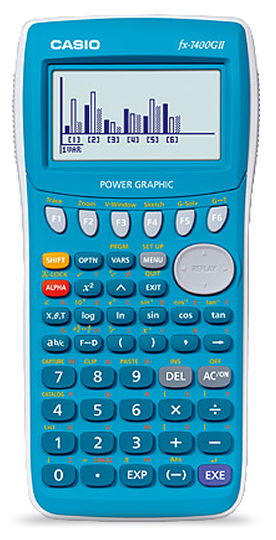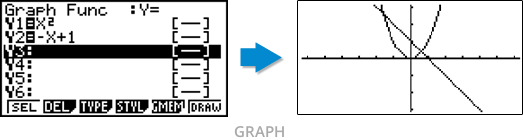Home > Products > Graphic Models > fx-7400GII

fx-7400GII

OverviewGraph Plotting Functions(GRAPH) that Deepen Understanding

Making it possible to specify the graph to plot for yourself serves to enhance your understanding of it, providing a better overall understanding of examples from the textbook.A Table Function(TABLE) that Demonstrates Regularity

Clearly laying out the stipulated function makes it much easier to gain a full understanding of it.A Variety of Equation Functions(EQUA)

Functions for quadratic to sextic equations and for simultaneous linear equations with 2-6 variables cover all of your basic equation needs, and allow for a full range of equation calculations.Other useful functions